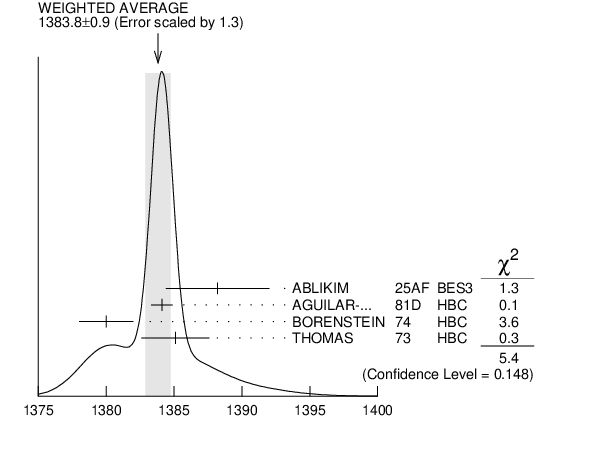# ${{\boldsymbol \Sigma}{(1385)}^{0}}$ MASS INSPIRE search

VALUE (MeV) EVTS DOCUMENT ID TECN  COMMENT
$\bf{ 1383.7 \pm1.0}$ OUR AVERAGE  Error includes scale factor of 1.4.
$1384.1$ $\pm0.8$ 5722
 1981 D
HBC ${{\mathit K}^{-}}$ ${{\mathit p}}$ $\rightarrow$ ${{\mathit \Lambda}}$3 ${{\mathit \pi}}$ 4.2 ${\mathrm {GeV/}}\mathit c$
$1380$ $\pm2$ 3100 1
 1974
HBC ${{\mathit K}^{-}}$ ${{\mathit p}}$ $\rightarrow$ ${{\mathit \Lambda}}$3 ${{\mathit \pi}}$ 2.18 ${\mathrm {GeV/}}\mathit c$
$1385.1$ $\pm2.5$ 240 2
 1973
HBC ${{\mathit \pi}^{-}}$ ${{\mathit p}}$ $\rightarrow$ ${{\mathit \Lambda}}{{\mathit \pi}^{0}}{{\mathit K}^{0}}$
• • • We do not use the following data for averages, fits, limits, etc. • • •
$1389$ $\pm3$ 500 3
 1979 B
HBC ${{\mathit K}^{-}}{{\mathit p}}$ 8.25 ${\mathrm {GeV/}}\mathit c$
1  From a fit to ${{\mathit \Lambda}}{{\mathit \pi}^{0}}$ with the width fixed at 34 MeV.
2  The error is enlarged to $\Gamma /\sqrt {\mathit N }$. See the note on the ${{\mathit K}^{*}{(892)}}$ mass in the 1984 edition.
3  From fit to inclusive ${{\mathit \Lambda}}{{\mathit \pi}^{0}}$ spectrum with the width fixed at 40 MeV.${{\mathit \Sigma}{(1385)}^{0}}$ mass (MeV)
References:
 AGUILAR-BENITEZ 1981D
AFIS A77 144 Study of Quasi Two-Body Processes with Production of ${{\mathit \Sigma}{(1385)}}$ in ${{\mathit \pi}}{{\mathit p}}$ and ${{\mathit K}^{-}}{{\mathit p}}$ Interactions at 4 GeV/$\mathit c$
 BAUBILLIER 1979B
NP B148 18 A Study of Inclusive ${{\mathit \Lambda}}$, ${{\mathit \Sigma}^{0}}$ and ${{\mathit \Sigma}{(1385)}^{0}}$ Production in ${{\mathit K}^{-}}{{\mathit p}}$ Interactions at 8.25 ${\mathrm {GeV/}}\mathit c$
 BORENSTEIN 1974
PR D9 3006 A Determination of the Mass, Width and the (${{\mathit \Sigma}}{{\mathit \pi}}/{{\mathit \Lambda}}{{\mathit \pi}}$) Branching Ratio of the ${{\mathit \Sigma}{(1381)}}$ Baryon
 THOMAS 1973
NP B56 15 Strange Particle Production from ${{\mathit \pi}^{-}}{{\mathit p}}$ Interactions at 1.69 ${\mathrm {GeV/}}\mathit c$